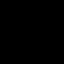Mathematics 70 OnlineOpenStudy (anonymous):

find the derivative of F(x)=(ln(3))^(cos(x))OpenStudy (anonymous):

$\ln(3)^{\cos(x)}$OpenStudy (anonymous):

yes.OpenStudy (anonymous):

Write the equation like $y = \ln(3)^{\cos(x)}$OpenStudy (anonymous):

-log(3)^(cos(x))*log(log(3))*sin(x)OpenStudy (anonymous):

I think....OpenStudy (anonymous):

i was thinking taking natural log on both sides but i dont think that would help??OpenStudy (anonymous):

yeah it won't because i'm not solving for x, i'm just trying to find the derivative.OpenStudy (anonymous):

i think it would.. lets tryOpenStudy (anonymous):

$\ln(y) = \cos(x) \ln(\ln(3))$OpenStudy (anonymous):

My answer is correct. The chain rule I used is a little overly complicated though.OpenStudy (anonymous):

$-\log(3)^{cosx}*(\log(\log(3))) *\sin(x)$OpenStudy (anonymous):

is that what you think it is?OpenStudy (anonymous):

u = cos(x); F'(x) = d(log^u(3))/du * du/dx; du/dx = -sin(x); d(log(3)^u)/du = log(3)^u * log(log(3)); F'(x) = log(3)^u * log(log(3)) * -sin(x)OpenStudy (agreene):

$\ln (\ln 3) -\sin (x) \ln^{cos(x)}(3)$OpenStudy (anonymous):

$ln(y) = -\ln(\ln(3))\sin(x)$OpenStudy (anonymous):

$\frac{y \prime}{y} = -\ln(\ln(3))\sin(x)$OpenStudy (anonymous):

$y \prime = y[-\ln(\ln(3))\sin(x)]$OpenStudy (anonymous):

$y \prime = \ln(3)^{\cos(x)}[-\ln(\ln(3))\sin(x)]$OpenStudy (anonymous):

Then i guess you would factor out the negative?OpenStudy (agreene):

I prefer my answer. I chain ruled it.OpenStudy (anonymous):

i'm confused on how you went from ln(y) to y'/yOpenStudy (anonymous):

the derivative of $\ln(y)$ is $\frac{1}{y} * y \prime$OpenStudy (anonymous):

i thought the derivative of ln(x)=(1/x)?OpenStudy (anonymous):

It's the chain rule of that function. the derivative of ln(x) = 1/x, so using the chain rule with u = y, d(ln(u))/du * du/dx = 1/u * y' = y'/u = y'/yOpenStudy (anonymous):

you have to use implicit differentiation because ln is a function of yOpenStudy (anonymous):

or y is a function of ln.. how ever it goesOpenStudy (anonymous):

y is a function of x.

Latest Questionsmoney207: slide in ma dmss -
13 minutes ago 3 Replies 0 Medalsmoney207: new friends?
21 minutes ago 6 Replies 1 Medallilbart: How many year will it be until its 2055
6 minutes ago 7 Replies 2 Medalssh2ah00d6: 3. For basketball fans, a flexible light source that can be attached around any basketball hoop rim has been developed.
1 hour ago 0 Replies 0 MedalsGucchi: english help
1 hour ago 14 Replies 1 MedalFlowerpower5290: Valkyrae drawing
47 minutes ago 19 Replies 10 MedalsAlreem7: Which of the following does no affect the frictional form? a.weight of the object b.
2 hours ago 2 Replies 1 MedalAlreem7: A car is moving on horizontal road with a constant velocity.What is the net force acting on the object? a.
3 hours ago 2 Replies 3 MedalsalphaXtiger: how is my story so far STALKER I am walking with my friend at night and I swear i see someone following me u201cBelladonna!u201dmy friend yells at me which
20 minutes ago 6 Replies 2 MedalsLILBOY: https://www.bandlab.com/post/4104d17c-3261-ee11-9937-000d3a41ec2a let me know wha
41 minutes ago 2 Replies 1 Medal